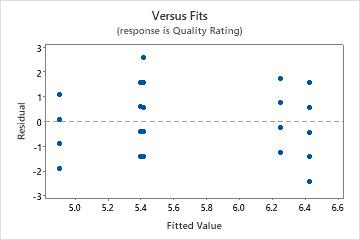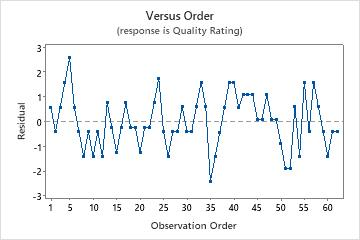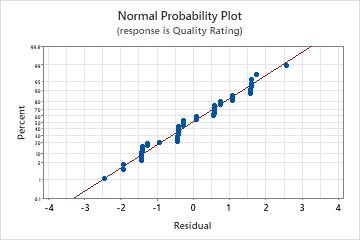# Interpret the key results for General MANOVA

Complete the following steps to interpret general MANOVA. Key output includes the p-value, the coefficients, R2, and the residual plots.

## Step 1: Test the equality of means from all the responses

To simultaneously test the equality of means from all the responses, compare the p-values in the MANOVA test tables for each term to your significance level. Usually, a significance level (denoted as α or alpha) of 0.05 works well. A significance level of 0.05 indicates a 5% risk of concluding that an association exists when there is no actual association.
P-value ≤ α: The differences between the means are statistically significant
If the p-value is less than or equal to the significance level, you can conclude that the differences between the means are statistically significant.
P-value > α: The differences between the means are not statistically significant
If the p-value is greater than the significance level, you cannot conclude that the differences between the means are statistically significant. You may want to refit the model without the term.
If there are multiple predictors without a statistically significant association with the response, you can reduce the model by removing terms one at a time. For more information on removing terms from the model, go to Model reduction.
If a model term is statistically significant, the interpretation depends on the type of term. The interpretations are as follows:
• If a main effect is significant, the level means for the factor are significantly different from each other across all responses in your model.
• If an interaction term is significant, the effects of each factor are different at each level of the other factors across all responses in your model. For this reason, you should not analyze the individual effects of terms involved in significant higher-order interactions.

## Step 2: Determine which response means have the largest differences for each factor

Use the eigen analysis to assess how the response means differ between the levels of the different model terms. You should focus on the eigenvectors that correspond to high eigenvalues. To display the eigen analysis, go to Stat > ANOVA > General MANOVA > Results and select Eigen analysis under Display of Results.

### EIGEN Analysis for Method

Eigenvalue 0.5848 0.00000 Proportion 1.0000 0.00000 Cumulative 1.0000 1.00000
Eigenvector 1 2 Usability Rating 0.144062 -0.07870 Quality Rating -0.003968 0.13976

## Step 3: Assess the differences between group means

Use the Means table to understand the statistically significant differences between the factor levels in your data. The mean of each group provides an estimate of each population mean. Look for differences between group means for terms that are statistically significant.

For main effects, the table displays the groups within each factor and their means. For interaction effects, the table displays all possible combinations of the groups. If an interaction term is statistically significant, do not interpret the main effects without considering the interaction effects.

To display the means, go to Stat > ANOVA > General MANOVA > Results, select Univariate analysis of variance, and enter the terms in Display least squares means corresponding to the terms.

Least Squares Means for Responses Usability Rating Quality Rating Mean SE Mean Mean SE Mean Method Method 1 4.819 0.1645 5.242 0.1932 Method 2 6.212 0.1794 6.026 0.2107 Plant Plant A 5.708 0.1924 5.833 0.2259 Plant B 5.493 0.2323 5.914 0.2727 Plant C 5.345 0.2059 5.155 0.2418 Method*Plant Method 1 Plant A 4.667 0.2721 5.417 0.3195 Method 1 Plant B 4.700 0.2981 5.400 0.3500 Method 1 Plant C 5.091 0.2842 4.909 0.3337 Method 2 Plant A 6.750 0.2721 6.250 0.3195 Method 2 Plant B 6.286 0.3563 6.429 0.4183 Method 2 Plant C 5.600 0.2981 5.400 0.3500

## Step 4: Assess the univariate results to examine individual responses

When you perform General MANOVA, you can choose to calculate the univariate statistics to examine the individual responses. The univariate results can provide a more intuitive understanding of the relationships in your data. However, the univariate results can differ from the multivariate results.

To display the univariate results, go to Stat > ANOVA > General MANOVA > Results and select Univariate analysis of variance under Display of Results.

To determine whether the association between the response and each term in the model is statistically significant, compare the p-value for the term to your significance level to assess the null hypothesis. The null hypothesis is that there is no association between the term and the response. Usually, a significance level (denoted as α or alpha) of 0.05 works well. A significance level of 0.05 indicates a 5% risk of concluding that an association exists when there is no actual association.
P-value ≤ α: The association is statistically significant
If the p-value is less than or equal to the significance level, you can conclude that there is a statistically significant association between the response variable and the term.
P-value > α: The association is not statistically significant
If the p-value is greater than the significance level, you cannot conclude that there is a statistically significant association between the response variable and the term. You may want to refit the model without the term.
If there are multiple predictors without a statistically significant association with the response, you can reduce the model by removing terms one at a time. For more information on removing terms from the model, go to Model reduction.
If a model term is statistically significant, the interpretation depends on the type of term. The interpretations are as follows:
• If a categorical factor is significant, you can conclude that not all the level means are equal.
• If an interaction term is significant, the relationship between a factor and the response depends on the other factors in the term. In this case, you should not interpret the main effects without considering the interaction effect.
• If a covariate is statistically significant, you can conclude that changes in the value of the covariate are associated with changes in the mean response value.
• If a polynomial term is significant, you can conclude that the data contain curvature.

## Step 5: Determine whether your model meets the assumptions of the analysis

Use the residual plots to help you determine whether the model is adequate and meets the assumptions of the analysis. If the assumptions are not met, the model may not fit the data well and you should use caution when you interpret the results.

When you perform General MANOVA, Minitab displays residual plots for all response variables that are in your model. You must determine whether the residual plots for all response variables indicate that the model meets the assumptions.

For more information on how to handle patterns in the residual plots, go to Residual plots for General MANOVA and click the name of the residual plot in the list at the top of the page.

### Residuals versus fits plot

Use the residuals versus fits plot to verify the assumption that the residuals are randomly distributed and have constant variance. Ideally, the points should fall randomly on both sides of 0, with no recognizable patterns in the points.

The patterns in the following table may indicate that the model does not meet the model assumptions.
Pattern What the pattern may indicate
Fanning or uneven spreading of residuals across fitted values Nonconstant variance
Curvilinear A missing higher-order term
A point that is far away from zero An outlier
A point that is far away from the other points in the x-direction An influential point
In this residuals versus fits plot, the data appear to be randomly distributed about zero. There is no evidence that the value of the residual depends on the fitted value.### Residuals versus order plot

Use the residuals versus order plot to verify the assumption that the residuals are independent from one another. Independent residuals show no trends or patterns when displayed in time order. Patterns in the points may indicate that residuals near each other may be correlated, and thus, not independent. Ideally, the residuals on the plot should fall randomly around the center line:If you see a pattern, investigate the cause. The following types of patterns may indicate that the residuals are dependent.
In this residuals versus order plot, the residuals appear to fall randomly around the centerline. There is no evidence that the residuals are not independent.### Normal probability plot of the residuals

Use the normal probability plot of the residuals to verify the assumption that the residuals are normally distributed. The normal probability plot of the residuals should approximately follow a straight line.

The patterns in the following table may indicate that the model does not meet the model assumptions.
Pattern What the pattern may indicate
Not a straight line Nonnormality
A point that is far away from the line An outlier
Changing slope An unidentified variable
In this normal probability plot, the points generally follow a straight line. There is no evidence of nonnormality, outliers, or unidentified variables.By using this site you agree to the use of cookies for analytics and personalized content.  Read our policy